## Recommended Books

### Filter books by:

 Strand NumberAlgebraMeasurementSpaceStatistics and ProbabilityProfessional ReadingProblem Solving Year Year 5Year 6Year 7Year 2Year 3Year 8Year 4Foundation YearYear 1Year 9Year 10Maths AMaths B Numeracy Strategy Multiplication and Division TriangleAct it OutPart-Part-WholeLook for a patternAdditive to Multiplicative ThinkingBenchmarkingSee the Parts to match the wholeNumber LineTables and GraphsDraw a diagramVisualisationFind the PatternDraw what you can't seeMental StrategiesVarious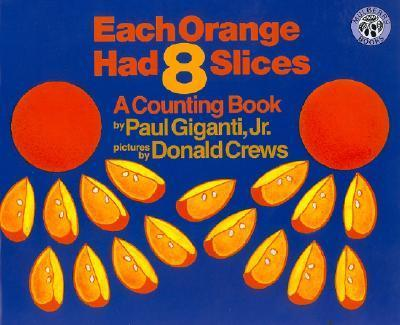## Each Orange Had 8 Slices

##### Australian Curriculum:  Description

F.Y – Establish understanding of the language and processes of counting by naming numbers in sequences, initially to and from 20, moving from  any starting point (ACMNA001); F.Y – Represent practical situations to model addition and sharing (ACMNA004); Year 1 – Represent and solve simple addition and subtraction problems using a range of strategies including counting on, partitioning and rearranging parts (ACMNA015); Year 3 – Recall multiplication facts of two, three, five and ten and related division facts (ACMNA056)

##### Teaching ideas
Build on the concept of counting on, partitioning and part-part-whole with other object sequences.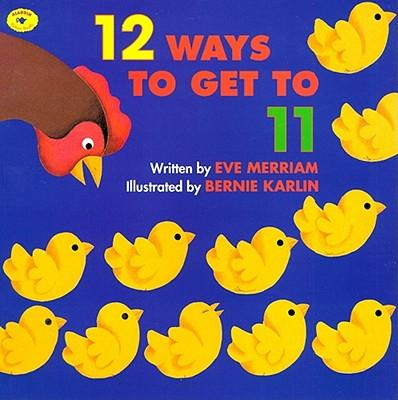## 12 Ways to Get to 11

##### Australian Curriculum:  Description

F.Y – Establish understanding of the language and processes of counting by naming numbers in sequences, initially to and from 20, moving from  any starting point (ACMNA001); F.Y – Represent practical situations to model addition and sharing (ACMNA004); Year 1 – Represent and solve simple addition and subtraction problems using a range of strategies including counting on, partitioning and rearranging parts (ACMNA015)

##### Teaching ideas
Build on the concept of counting on, partitioning and part-part-whole with other object sequences.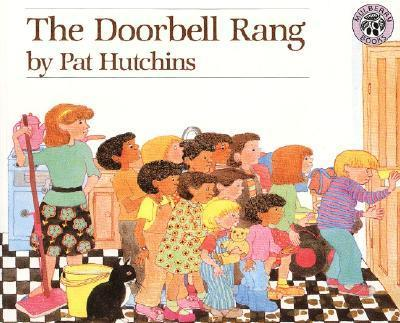## The Doorbell Rang

##### Australian Curriculum:  Description

Number and Algrbra – Number and Place Value – Develop efficient mental and written strategies and use appropriate digital technologies for multiplication and for division where there is no remainder (ACMNA076)

##### Teaching ideas
Students could investigate other numbers that divide equally. What if the original batch had been 24/36/48. How would that have been shared between the guests (2, 4, 6, 12)## Sir Cumference and the Sword in the Cone

##### Australian Curriculum:  Description

Year 5 – Measurement and Geometry : Shape – Connect three-dimensional objects with their nets and other two-dimensional representations (ACMMG111); Year 6 – Measurement and Geometry : Shape – Construct simple prisms and pyramids (ACMMG140); Year 7 – Measurement and Geometry : Shape – Draw different views of prisms and solids formed from combinations of prisms (ACMMG161); Year 8 – Measurement and Geometry : Shape – Investigate the relationship between features of circles such as circumference, area, radius and diameter. Use formulas to solve problems involving circumference and area (ACMMG197).

##### Teaching ideas
* Year 5 students could use page 5 to "form the solids and find their places"; * Year 6 students could use page 5 to "form the solids and find their places"; * Year 7 students could investigate Euler's Law by folding nets to form prisms and pyramids. They =could then draw up an investigation table (page 12) and draw their own conclusions; * By year 8 the mathematical vocabulary students know should enable them to understand and apprecaite the entire book. The teacher could pose the problem from the book without reading it, and have students work in groups to solve it. Were the steps you took to solving it the same as Radius and Vertex's?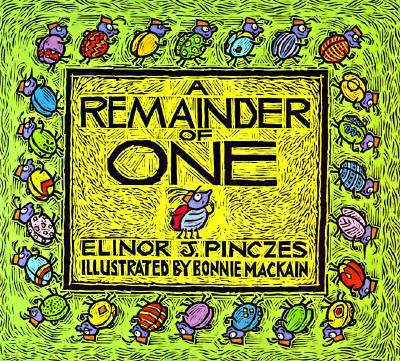## A Remainder of One

##### Australian Curriculum:  Description

Number and Algebra : Number and Place Value – Solve problems involving division by a one digit number, including those that result in a remainder (ACMNA101); Investigate index notation and represent whole numbers as products of powers of prime numbers (ACMNA149)

##### Teaching ideas
* Have students mathematically represent the ideas in the book. i.e. concrete with actual bugs, representational with tally marks and abstract would be the mathematical numbers and symbols (25 ÷ 2 = 12 r1; 25 ÷ 3 = 8 r1; 25 ÷ 4 = 6 r1; 25 ÷ 5 = 5). Year 7s could use this as an introduction to prime numbers and non-prime numbers. What are the factors of 24 and 25.## Divide & Ride

##### Australian Curriculum:  Description

Solve problems involving division by a one digit number, including those that result in a remainder (ACMNA101)

##### Teaching ideas
"Student investigate other ""Total Amounts"". How many people can go on the ride? What is the best number of people to go to the fair so that there is nobody sitting alone?"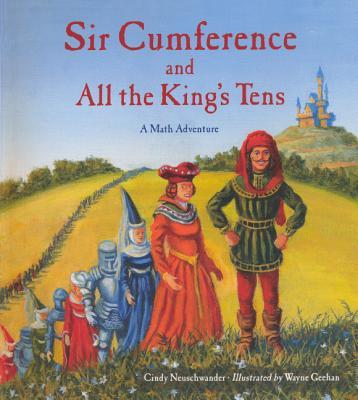## Sir Cumference and All the King’s Tens

##### Australian Curriculum:  Description

2-Recognise, model, represent and order numbers to at least 1000 (ACMNA027); 2-Group, partition and rearrange collections up to 1000 in hundreds, tens and ones to facilitate more efficient counting (ACMNA028); 3-Recognise, model, represent and order numbers to at least 10 000 (ACMNA052); 3-Apply place value to partition, rearrange and regroup numbers to at least 10 000 to assist calculations and solve problems (ACMNA053)

##### Teaching ideas
Other place value sheets, activities, games, representations